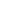Double Digit Subtraction Worksheets (With Borrowing)

Worksheets of double digit subtraction (with borrowing)

Double Digit Subtraction Worksheets (No Borrowing)

Worksheets of double digit subtraction (no borrowing)

Single Digit Simple Subtraction Worksheets

Worksheets of single digit subtraction

Visual Subtraction Worksheets

Worksheets of visual subtraction

Picture Subtraction Worksheets

Worksheets of subtracting using pictures